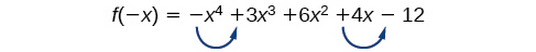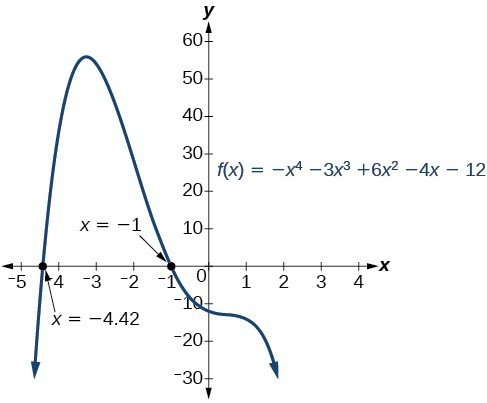## Use Descartes’ Rule of Signs

There is a straightforward way to determine the possible numbers of positive and negative real zeros for any polynomial function. If the polynomial is written in descending order, Descartes’ Rule of Signs tells us of a relationship between the number of sign changes in $f\left(x\right)$ and the number of positive real zeros. For example, the polynomial function below has one sign change.

This tells us that the function must have 1 positive real zero.

There is a similar relationship between the number of sign changes in $f\left(-x\right)$ and the number of negative real zeros.

In this case, $f\left(\mathrm{-x}\right)$ has 3 sign changes. This tells us that $f\left(x\right)$ could have 3 or 1 negative real zeros.

### A General Note: Descartes’ Rule of Signs

According to Descartes’ Rule of Signs, if we let $f\left(x\right)={a}_{n}{x}^{n}+{a}_{n - 1}{x}^{n - 1}+…+{a}_{1}x+{a}_{0}$ be a polynomial function with real coefficients:

• The number of positive real zeros is either equal to the number of sign changes of $f\left(x\right)$ or is less than the number of sign changes by an even integer.
• The number of negative real zeros is either equal to the number of sign changes of $f\left(-x\right)$ or is less than the number of sign changes by an even integer.

### Example 7: Using Descartes’ Rule of Signs

Use Descartes’ Rule of Signs to determine the possible numbers of positive and negative real zeros for $f\left(x\right)=-{x}^{4}-3{x}^{3}+6{x}^{2}-4x - 12$.

### Solution

Begin by determining the number of sign changes.

There are two sign changes, so there are either 2 or 0 positive real roots. Next, we examine $f\left(-x\right)$ to determine the number of negative real roots.

$\begin{cases}f\left(-x\right)=-{\left(-x\right)}^{4}-3{\left(-x\right)}^{3}+6{\left(-x\right)}^{2}-4\left(-x\right)-12\hfill \\ f\left(-x\right)=-{x}^{4}+3{x}^{3}+6{x}^{2}+4x - 12\hfill \end{cases}$Again, there are two sign changes, so there are either 2 or 0 negative real roots.

There are four possibilities, as we can see below.

Positive Real
Zeros
Negative Real
Zeros
Complex
Zeros
Total
Zeros
2 2 0 4
2 0 2 4
0 2 2 4
0 0 4 4

### Analysis of the Solution

We can confirm the numbers of positive and negative real roots by examining a graph of the function. We can see from the graph in Figure 3 that the function has 0 positive real roots and 2 negative real roots.Figure 3

### Try It 6

Use Descartes’ Rule of Signs to determine the maximum possible numbers of positive and negative real zeros for $f\left(x\right)=2{x}^{4}-10{x}^{3}+11{x}^{2}-15x+12$. Use a graph to verify the numbers of positive and negative real zeros for the function.

Solution